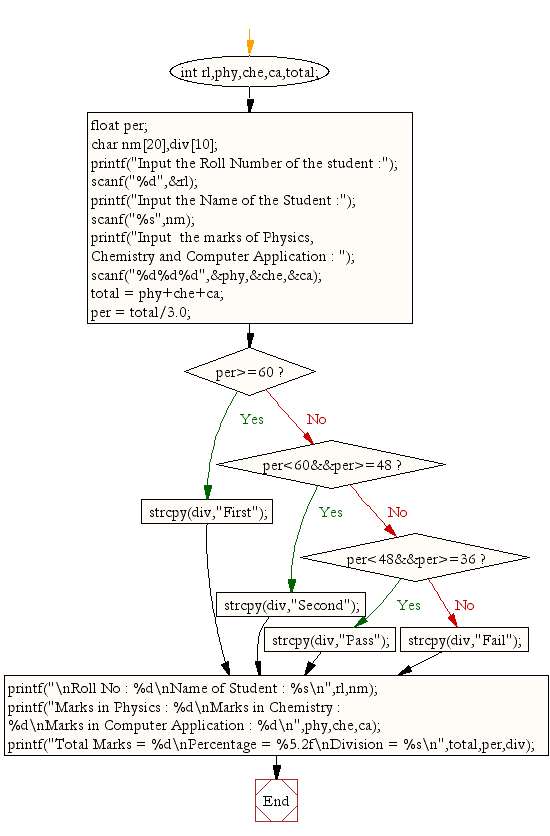﻿ C : Calculate the total, percentage of marks for 3 subjects# C Exercises: Calculate the total, percentage and division to take marks of three subjects

## C Conditional Statement: Exercise-12 with Solution

Write a C program to read roll no, name and marks of three subjects and calculate the total, percentage and division.

Sample Solution:

C Code:

``````#include <stdio.h>
#include <string.h>

void main()
{
int rl,phy,che,ca,total;
float per;
char nm,div;
printf("Input the Roll Number of the student :");
scanf("%d",&rl);
printf("Input the Name of the Student :");
scanf("%s",nm);
printf("Input  the marks of Physics, Chemistry and Computer Application : ");
scanf("%d%d%d",&phy,&che,&ca);
total = phy+che+ca;
per = total/3.0;
if (per>=60)
strcpy(div,"First");
else
if (per<60&&per>=48)
strcpy(div,"Second");
else
if (per<48&&per>=36)
strcpy(div,"Pass");
else
strcpy(div,"Fail");

printf("\nRoll No : %d\nName of Student : %s\n",rl,nm);
printf("Marks in Physics : %d\nMarks in Chemistry : %d\nMarks in Computer Application : %d\n",phy,che,ca);
printf("Total Marks = %d\nPercentage = %5.2f\nDivision = %s\n",total,per,div);
}
```
```

Sample Output:

```Input the Roll Number of the student :784
Input the Name of the Student :James
Input  the marks of Physics, Chemistry and Computer Application : 70 80 90

Roll No : 784
Name of Student : James
Marks in Physics : 70
Marks in Chemistry : 80
Marks in Computer Application : 90
Total Marks = 240
Percentage = 80.00
Division = First
```

Flowchart:C Programming Code Editor:

Improve this sample solution and post your code through Disqus.

What is the difficulty level of this exercise?

Test your Programming skills with w3resource's quiz.

﻿

## C Programming: Tips of the Day

Why do C and C++ compilers allow array lengths in function signatures when they're never enforced?

It is a quirk of the syntax for passing arrays to functions.

Actually it is not possible to pass an array in C. If you write syntax that looks like it should pass the array, what actually happens is that a pointer to the first element of the array is passed instead.

Since the pointer does not include any length information, the contents of your [] in the function formal parameter list are actually ignored.

Ref : https://bit.ly/3fhlvdH